# ANALYSIS OF GRAVITY DAMReading time: 1 minute

The stability of a dam can be analysed in the following steps:i. Consider unit length of the dam.

ii. Work out the magnitude and dimensions of all the vertical forces acting on the dam and their algebraic sum, i.e. ?V.

iii. Similarly work out all the horizontal forces and their algebraic sum i.e. ?H.

iv. Determine the lever arm of all these forces above the toe.

v. Determine the moments of these forces about the toe and find the algebraic sum of all those moments, i.e. ?M.

vi. Find out the location of the resulting force by determining its distance from the toe.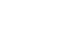vii. Find out the eccentricity (e) of the resultant (R) using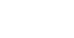It must be less than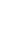in order to ensure that no tension is developed anywhere in the dam.

viii. Determine the vertical stresses at the toe and heel using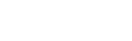ix. Determine the maximum normal stresses.

x. Determine the factor of safety against overturning as equal to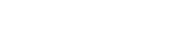xi. Determine the factor of safety against sliding using

Sliding factor =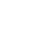Shear friction factor (S.F.F)

=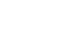See here Structure of a Gravity Dam